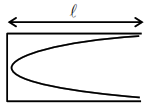# A tuning fork is vibrating`
Question:

A tuning fork is vibrating at $250 \mathrm{~Hz}$. The length of the shortest closed organ pipe that will resonate with the tuning fork will be $\mathrm{cm}$.

(Take speed of sound in air as $340 \mathrm{~ms}^{-1}$ )

Solution:$\frac{\lambda}{4}=\ell \Rightarrow \lambda=4 \ell$

$\mathrm{f}=\frac{\mathrm{V}}{\lambda}=\frac{\mathrm{V}}{4 \ell}$

$\Rightarrow 250=\frac{340}{4 \ell}$

$\Rightarrow \ell=\frac{34}{4 \times 25}=0.34 \mathrm{~m}$

$\ell=34 \mathrm{~cm}$